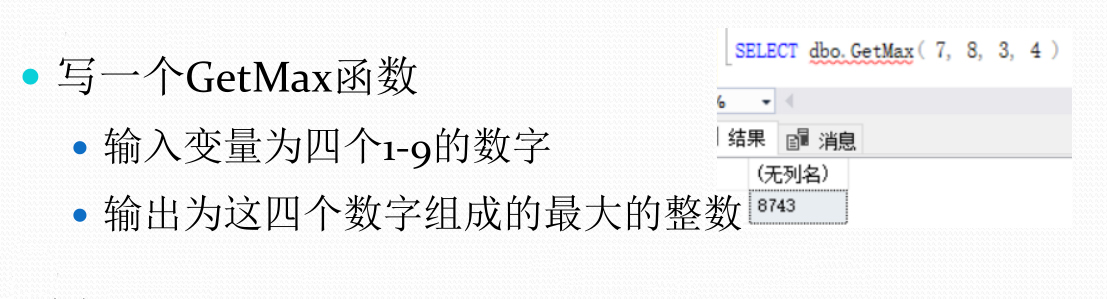SQL/TSQL 存储过程和函数 输出组成的最大数CREATE FUNCTION GetMax(
@a int,
@b int,
@c int,
@d int
)
RETURNS INT
AS
BEGIN
？？？？？
END

SELECT dbo.GetMax(7,8,3,4)

2个回答

CREATE FUNCTION GetMax(@a int,@b int,@c int,@d int)
RETURNS INT
AS

BEGIN
declare @str varchar(4000)
declare @tmp table(n varchar(100))
set @str =''

``````insert into @tmp
select @a union all
select @b union all
select @c union all
select @d

SELECT @str = @str + n FROM @tmp order by n desc
RETURN CAST(@str AS INT)
``````

END

--SELECT dbo.GetMax(7,8,3,4)

CREATE FUNCTION GetMax(
@a int,
@b int,
@c int,
@d int
)
RETURNS int
AS
BEGIN
declare @max int
set @max=@a
if @max<@b
set @max=@b
if @max<@c
set @max=@c
if @max<@d
set @max=@d
return @max
ENDfreedomxa007 回复hu15889920913: create FUNCTION GetMax(@a int,@b int,@c int,@d int ) RETURNS int AS BEGIN declare @max varchar(10) declare @tb table(num int) insert into @tb select @a union all select @b union all select @c union all select @d select @max=isnull(@max+',','')+convert(varchar(10),num)from @tb order by num desc return convert(int,replace(@max,',','')) END 刚刚没看清，这样就可以了